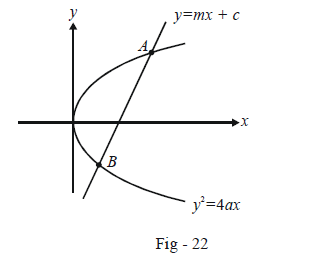# Basic Examples on Parabolas Set 3

Go back to  'Parabola'

Example – 12

A circle on any focal chord of a parabola as diameter cuts the curve again in P and Q. Show that PQ passes through a fixed point.

Solution: In example -8, we wrote the equation of a circle described on any focal chord as diameter in terms of one of its end points $${t_0}$$.

$(x - at_0^2)\left( {x - \frac{a}{{t_0^2}}} \right) + (y - 2a{t_0})\left( {y + \frac{{2a}}{{{t_0}}}} \right) = 0 \qquad\qquad\qquad \dots \dots \left( 1 \right)$

The intersection of this circle with the parabola can be evaluated by using the parametric form of the parabola :

$x = a{t^2},\,\,y = 2at \qquad\qquad\qquad\qquad\qquad \dots\dots \left( 2 \right)$

Using (2) in (1), we obtain

\begin{align}&{a^2}({t^2} - t_0^2)\left( {{t^2} - \frac{1}{{t_0^2}}} \right) + 4{a^2}(t - {t_0})\left( {t + \frac{1}{{{t_0}}}} \right) = 0\\& \Rightarrow {t^4} + {t^2}\left( {4 - t_0^2 - \frac{1}{{t_0^2}}} \right) - 4t\left( {{t_0} - \frac{1}{{{t_0}}}} \right) - 3 = 0 \qquad \qquad\qquad\dots \left( 3 \right) \end{align}

This has four roots in t, meaning that the circle intersects the parabola in four points, two of which are obviously $${t_0}$$ and \begin{align}- \frac{1}{{{t_0}}}\end{align} (since these are the points using which the circle has been described in the first place !).

Let the other two point P and Q be $${t_1}\;and{\text { }}{t_2}$$. Thus {t_0},\,\, - \begin{align}\frac{1}{{{t_0}}}\end{align},\,\,{t_1},\,\,{t_2} are the roots of (3):

\begin{align}& \qquad \;\;{t_0} + \left( { - \frac{1}{{{t_0}}}} \right) + {t_1} + {t_2} = 0\,\,\,;\,\,\,{t_0} \cdot \left( { - \frac{1}{{{t_0}}}} \right) \cdot {t_1} \cdot {t_2} = - 3\\& \Rightarrow \qquad {t_1} + {t_2} = \frac{1}{{{t_0}}} - {t_0}{\rm{ }}\,and \,{\rm{ }}{t_1}{t_2} = 3 \qquad \qquad \qquad \qquad\qquad\qquad\dots\left( 4 \right)\end{align}

The co-ordinates of P and Q are $$(at_1^2,\,\,2a{t_1}){\rm{ \, and}} \, {\rm{ }}(at_2^2,\,\,2a{t_2}).$$ Thus, the equation of PQ becomes :

\begin{align}& \qquad \quad \;\;PQ:\frac{{y - 2a{t_1}}}{{x - at_1^2}} = \frac{{2a({t_1} - {t_2})}}{{a(t_1^2 - t_2^2)}}\\&\Rightarrow \qquad ({t_1} + {t_2})y = 2x + 2a{t_1}{t_2}\qquad \qquad\qquad\qquad \dots \left( 5 \right)\end{align}

Using (4) in (5), we obtain

$\left( {\frac{1}{{{t_0}}} - {t_0}} \right)y = 2x + 6a$

This is always satisfied by (–3a, 0), no matter what the value of $${t_0}$$ may be. Thus, PQ always passes through the fixed point (–3a, 0).

Example - 13

Find the length of the intercept that the parabola $${y^2} = 4ax$$ makes on the line $$y = mx + c.$$

Solution: Let the end-points of the intercept be A and B :The co-ordinates of A and B can be evaluated by simultaneously solving the equation of the line and the parabola :

\begin{align}&\qquad\quad \;\;y = mx + c\\\\&\qquad \quad \;\;{y^2} = 4ax\\\\& \Rightarrow \qquad {(mx + c)^2} = 4ax\\\\ &\Rightarrow \qquad {m^2}{x^2} + (2mc - 4a)x + {c^2} = 0 \qquad \qquad \dots \left( 1 \right)\end{align}

The first point to be noted from this quadratic is that the line will intersect the parabola in two distinct points only if the D of (1) is positive, because only then will two distinct values of x be obtained (the case when $$m = \infty$$ gives two distinct points of intersection for one value of x, but that can be considered separately; in that case, the quadratic (1) will not be formed). We thus have,

\begin{align}& \qquad\qquad D > 0\\\\& \Rightarrow \qquad{{{(mc - 2a)}^2} > {m^2}{c^2}}\\\\&\ \Rightarrow \qquad {4amc < 4{a^2}} \\\\&\Rightarrow \qquad \boxed{mc < a} \qquad \qquad (a \text{ is +ve here)}\end{align}

Thus, the line will intersect the parabola only if a > mc. If a = mc, the line will be a tangent to the parabola. If a < cm, the line will not intersect the circle at all.

Assuming that D > 0, suppose that the roots of (1) are $${x_1}\;and\;{\text{ }}{x_2}:$$

\begin{align}&x + {x_2}= \frac{{4a - 2mc}}{{{m^2}}},\,\,{x_1}{x_2} = \frac{{{c^2}}}{{{m^2}}}\\&\!\!\!\!\!\!\!\!\!\!\!\!\!\!\!\!\!\!\!\!\!\!\!\Rightarrow \!\!\quad {({x_1} - {x_2})^2} = {({x_1} + {x_2})^2} - 4{x_1}{x_2}\\\\&\qquad\;\;\;= \frac{{{{(4a - 2mc)}^2}}}{{{m^4}}} - \frac{{4{c^2}}}{{{m^2}}}\\\\& \qquad\;\;\;= \frac{{16{a^2} + 4{m^2}{c^2} - 16amc - 4{m^2}{c^2}}}{{{m^4}}}\\\\ &\qquad\;\;\;= \frac{{16a(a - mc)}}{{{m^4}}}\end{align}

The corresponding y co-ordinates for $${x_1}\,and \,{\text{ }}{x_2}$$  are

${{y}_{1}}=m{{x}_{1}}+c\text{ }and~\text{ }{{y}_{2}}=m{{x}_{2}}+c$

so that $${y_2} - {y_1} = m({x_2} - {x_1})$$ .

The length AB (intercept) is now simply

\begin{align}AB &= \sqrt {{{({x_1} - {x_2})}^2} + {{({y_1} - {y_2})}^2}} \\\\ &= \sqrt {{{({x_1} - {x_2})}^2} + {m^2}{{({x_1} - {x_2})}^2}} \\\\ &= \frac{{4\sqrt {a(a - mc)} }}{{{m^2}}} \cdot \sqrt {1 + {m^2}} \end{align}

This result need not be remembered. What is important is that you understand the underlying approach.

From this example, we can deduce one more useful thing : for a non-zero variable $$m \in \mathbb{R},$$ the line y = mx + \begin{align}\frac{a}{m}\end{align} will always be a tangent to the circle. Verify that the point of contact of this tangent with the parabola is \begin{align}\left( {\frac{a}{{{m^2}}},\frac{{2a}}{m}} \right).\end{align} We’ll discuss tangents in more detail in the next section.